# Spherically Symmetric Configurations

## Principal Governing Equations

If the self-gravitating configuration that we wish to construct is spherically symmetric, then the coupled set of multidimensional, partial differential equations that serve as our principal governing equations can be simplified to a coupled set of one-dimensional, ordinary differential equations. This is accomplished by expressing each of the multidimensional spatial operators — gradient (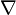$\nabla$), divergence (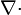$\nabla\cdot$), and Laplacian (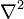$\nabla^2$) — in spherical coordinates (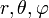$r, \theta, \varphi$) (see, for example, the Wikipedia discussion of integration and differentiation in spherical coordinates) then setting to zero all derivatives that are taken with respect to the angular coordinates θ and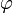$\varphi$. After making this simplification, our governing equations become,

Equation of Continuity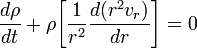$\frac{d\rho}{dt} + \rho \biggl[\frac{1}{r^2}\frac{d(r^2 v_r)}{dr} \biggr] = 0$

Euler Equation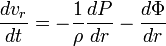$\frac{dv_r}{dt} = - \frac{1}{\rho}\frac{dP}{dr} - \frac{d\Phi}{dr}$

First Law of Thermodynamics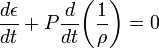$~\frac{d\epsilon}{dt} + P \frac{d}{dt} \biggl(\frac{1}{\rho}\biggr) = 0$

Poisson Equation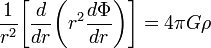$\frac{1}{r^2} \biggl[\frac{d }{dr} \biggl( r^2 \frac{d \Phi}{dr} \biggr) \biggr] = 4\pi G \rho$

## Structure

Equilibrium, spherically symmetric structures are obtained by searching for time-independent solutions to the above set of simplified governing equations. The steady-state flow field that must be adopted to satisfy both a spherically symmetric geometry and the time-independent constraint is,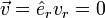$\vec{v} = \hat{e}_r v_r = 0$. After setting the radial velocity, vr, and all time-derivatives to zero, we see that the 1st (continuity) and 3rd (first law of thermodynamics) equations are trivially satisfied while the 2nd (Euler) and 4th give, respectively,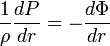$\frac{1}{\rho}\frac{dP}{dr} =- \frac{d\Phi}{dr}$ ,

and,$\frac{1}{r^2} \biggl[\frac{d }{dr} \biggl( r^2 \frac{d \Phi}{dr} \biggr) \biggr] = 4\pi G \rho$ .

(We recognize the first of these expressions as being the statement of hydrostatic balance appropriate for spherically symmetric configurations.)

We need one supplemental relation to close this set of equations because there are two equations, but three unknown functions —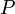$~P$(r),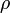$~\rho$(r), and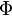$~\Phi$(r). As has been outlined in our discussion of supplemental relations for time-independent problems, in the context of this H_Book we will close this set of equations by specifying a structural, barotropic relationship between$~P$ and$~\rho$. (See below.)

### Solution Strategies

In an accompanying discussion, we outline three different techniques that have been used to solve the above pair of differential equations analytically, or to set them up for numerical solution once a supplemental barotropic relation has been identified. In summary, these techniques lead to the following governing relations:

#### Technique #1

This technique results in the following integro-differential equation which only depends on the two unknown functions,$~P$ and$~\rho$: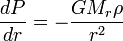$~\frac{dP}{dr} = - \frac{GM_r \rho}{r^2}$

where,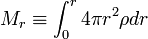$M_r \equiv \int_0^r 4\pi r^2 \rho dr$ .

#### Technique #2

This technique results in the following second-order differential equation which depends only on the two unknown functions,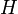$~H$ and$~\rho$: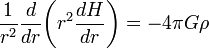$\frac{1}{r^2} \frac{d}{dr}\biggl( r^2 \frac{dH}{dr} \biggr) =- 4\pi G \rho$ .

#### Technique #3

This technique does not immediately reduce the number of equations nor the number unknown variables, but it does change one of the governing differential equations into an algebraic relation. The resulting pair of equations are,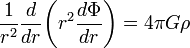$\frac{1}{r^2} \frac{d }{dr} \biggl( r^2 \frac{d \Phi}{dr} \biggr) = 4\pi G \rho$ ,

and

H + Φ = constant ;

the unknown functions are$~H$,$~\rho$, and$~\Phi$.

## Stability & Dynamics

 SUMMARY: The equilibrium structure of a self-gravitating, non-rotating, uniform-density sphere can be described analytically. The equilibrium structure of a self-gravitating, non-rotating, uniform-density sphere can be described analytically. The equilibrium structure of a self-gravitating, non-rotating, uniform-density sphere can be described analytically. The equilibrium structure of a self-gravitating, non-rotating, uniform-density sphere can be described analytically. The equilibrium structure of a self-gravitating, non-rotating, uniform-density sphere can be described analytically. The equilibrium structure of a self-gravitating, non-rotating, uniform-density sphere can be described analytically. The equilibrium structure of a self-gravitating, non-rotating, uniform-density sphere can be described analytically. SUMMARY: The equilibrium structure of a self-gravitating, non-rotating, uniform-density sphere can be described analytically. The equilibrium structure of a self-gravitating, non-rotating, uniform-density sphere can be described analytically. The equilibrium structure of a self-gravitating, non-rotating, uniform-density sphere can be described analytically. The equilibrium structure of a self-gravitating, non-rotating, uniform-density sphere can be described analytically. The equilibrium structure of a self-gravitating, non-rotating, uniform-density sphere can be described analytically. The equilibrium structure of a self-gravitating, non-rotating, uniform-density sphere can be described analytically. The equilibrium structure of a self-gravitating, non-rotating, uniform-density sphere can be described analytically.

 © 2014 - 2020 by Joel E. Tohline |   H_Book Home   |   YouTube   | Appendices: | Equations | Variables | References | Ramblings | Images | myphys.lsu | ADS |